At XDC 2016 there was a lot of interest in our Compiler session and LLVM. I’ve summarized a bit about LLVM in an earlier post, but to take things further, we put together this series of blog posts on compilers.

These will all be at a high-level. None of these posts are going to teach you how to write a compiler. The goal of these posts is for you to have a basic understanding of the components of a compiler and how they all work together to create a native app.

This is the second post in our ongoing series on compilers. I recommend that you first read Compilers 101 – Overview and Lexer before continuing.

After the Lexer has converted your source code to tokens, it sends them to the Parser. The job of the Parser is to turn these tokens into abstract syntax trees, which are representations of the source code and its meaning.

For reference, this is the simple source code we are “compiling”as we go through the parts of the compiler:

`sum = 3.14 + 2 * 4`

The lexer has converted this to a stream of tokens which are now sent to the Parser to process. The tokens are:

1. Type: identifier
1. value: sum
2. start: 0
3. length: 3
2. Type: equals or assigns
1. value: n/a
2. start: 4
3. length: 1
3. Type: double
1.  value: 3.14
2.  start: 6
3. length: 4
4. Type: plus
1. value: n/a
2. start: 11
3. length: 1
5. Type: integer
1. value: 2
2. start: 15
3. length: 1
6. Type: multiply
1. value: n/a
2. start: 15
3. length: 1
7. Type: integer
1. value: 4
2. start: 17
3. length: 1

## Parser

To see how this works, we’ll go through the tokens and create the syntax tree.

The first token is the identifier, which the parser knows is actually a variable. So it becomes the first node of the tree:The next token is equals or assigns. The parser knows things about this such as that it is an assignment and that assignment is a binary operator that has two operands, one on the left and one on the right. The variable from above is the left value, so it gets moved to the left side of the Assignment node that is now added to the tree to look like this: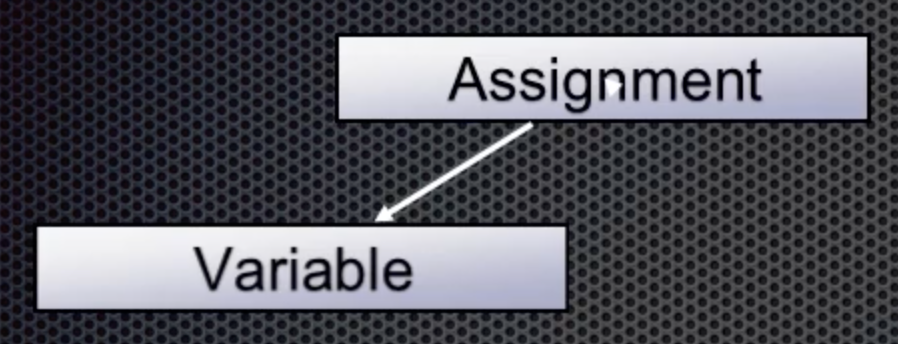Continuing, the double token is next with value 3.14. This is the right value for the assignment: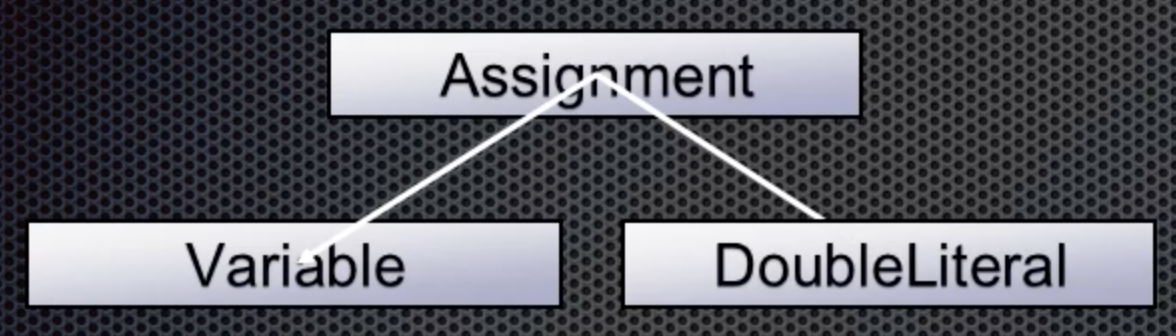Moving along, the plus token is next. The parser knows this is the addition operator (BinaryOperator+) that takes two values (and is also left associative). This means that the addition is added to the tree with the double as its left value: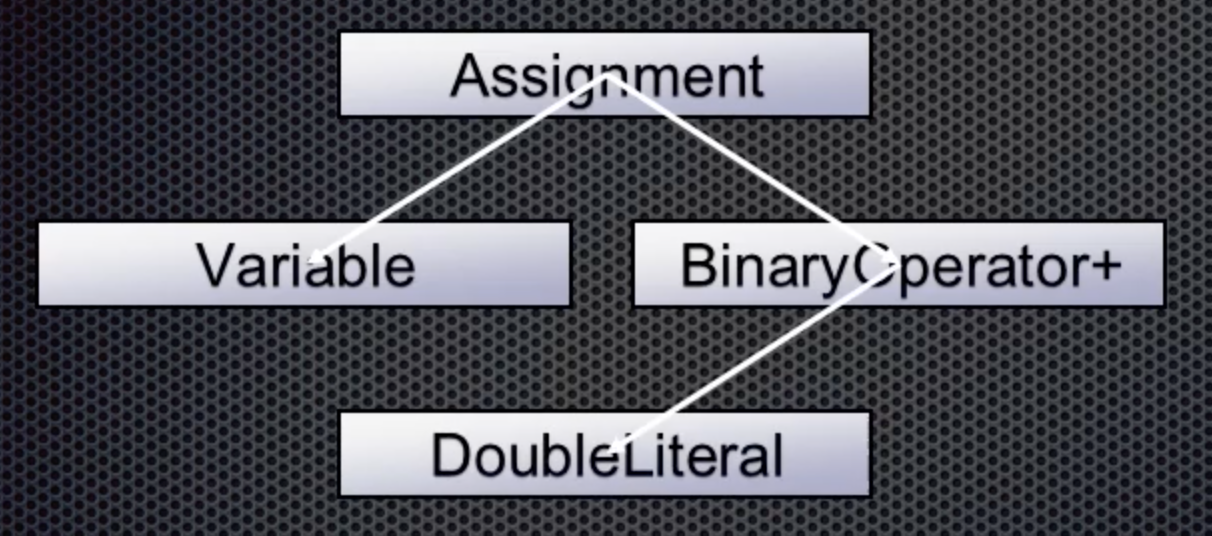After the plus token, an integer is next and this becomes the right value for the BinaryOperator+ node: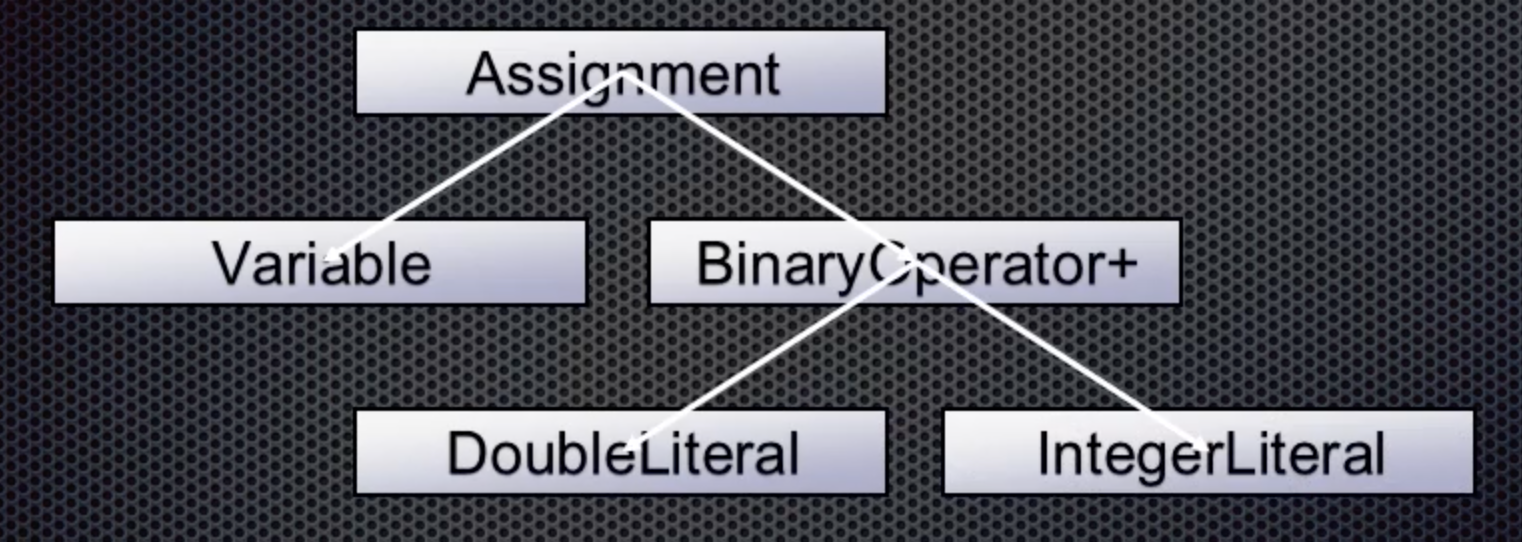Next is the multiply token, another binary operator that is left associative. So it gets the integer as its left value: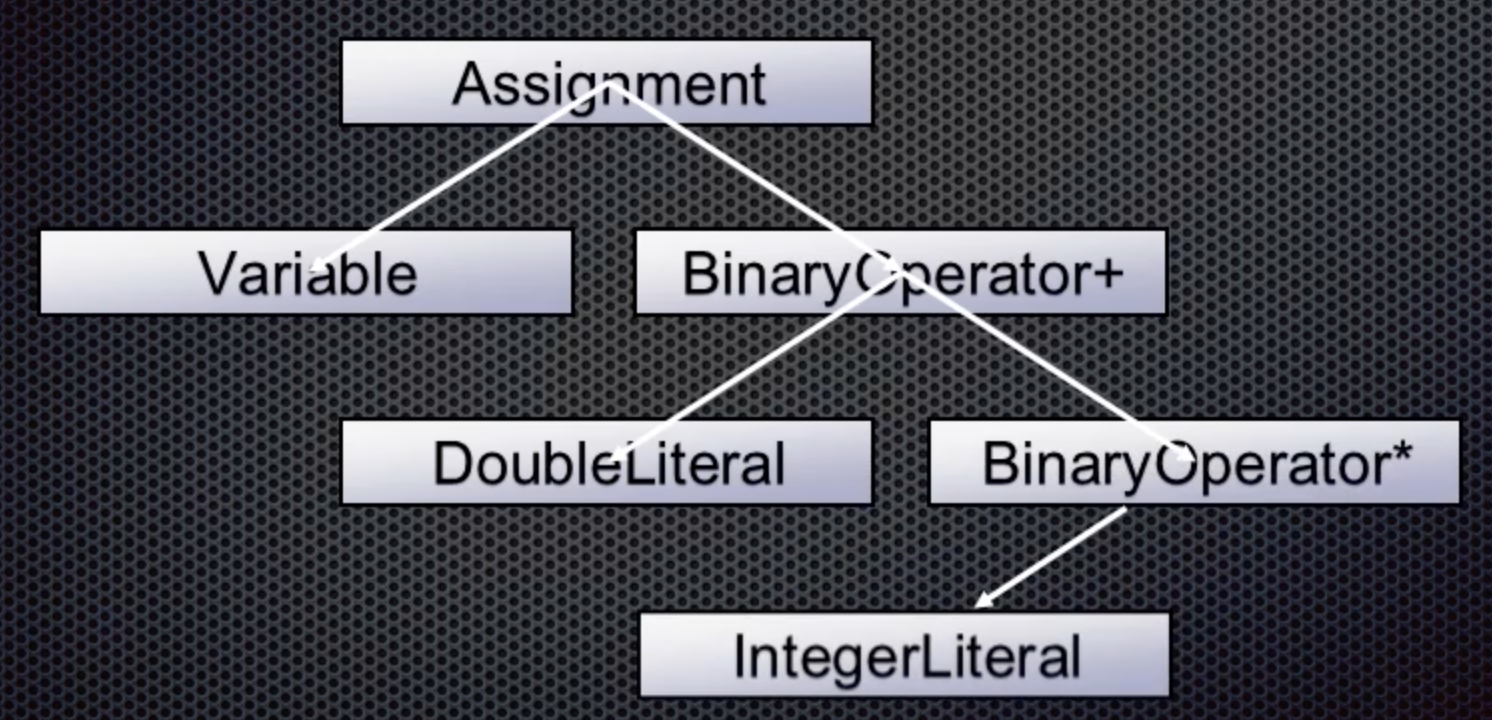The last token is another integer which becomes the right value for the multiplication: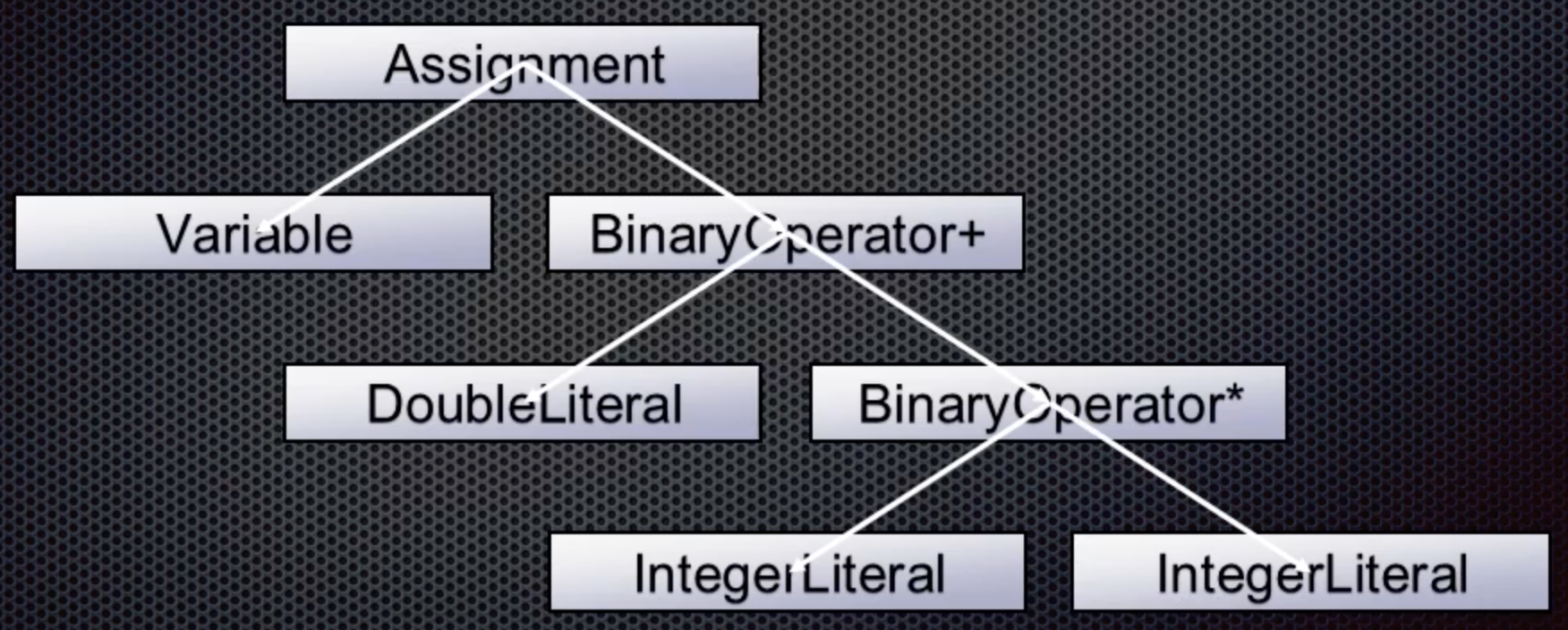And that is the final abstract syntax tree for our simple line of code. The Parser has done its work and has now created a tree that no longer represents the exact source code but is an idealized representation of what the user wrote.

This tree is then provided to the next component, the Semantic Analyzer.